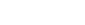# Interference and System Capacity

Interference is the major limiting factor in the performance of cellular radio systems.

Sources of interference:
There are two types of sources of interference:
a. Active

b. Passive

a. Active
Active interference sources that emits a radio signals on the same frequency.
1. another mobile in the same cell.
2. a call in progress in a neighboring cell.
3. other base stations operating in the same frequency band, or any noncellular system which inadvertently leaks energy into the cellular frequency band.

b. Passive
Passive source of wireless interference is any substance that restricts to degrade a wireless signal that attempt to pass through it.
Examples of such substances are metal, concrete, paper, fabric, glass, stone wood, bricks, etc..

Problems because of interference:
1. Interference on voice channels causes cross talk, where the subscriber hears interference in the background due to an undesired transmission.
2. On control channels, interference leads to missed and blocked calls due to errors in the digital signaling.

Interference is more severe in urban areas, due to the greater RF noise floor and the large number of base stations and mobiles. Interference has been recognized as a major bottleneck in increasing capacity and is often responsible for dropped calls.

Types of interference:
The two major types of system-generated cellular interference are co-channel interference and adjacent channel interference
Even though interfering signals are often generated within the cellular system, they are difficult to control in practice (due to random propagation effects). Even more difficult to control is interference due to out-of-band users, which arises without warning due to front end overload of subscriber equipment or intermittent intermodulation products. In practice, the transmitters from competing cellular carriers are often a significant source of out-of-band interference, since competitors often locate their base stations in close proximity to one another in order to provide comparable coverage to customers.

1. Co-channel Interference and System Capacity

Frequency reuse implies that in a given coverage area there are several cells that use the same set of frequencies. These cells are called co-channel cells, and the interference between signals from these cells is called co-channel interference. Unlike thermal noise which can be overcome by increasing the signal-to-noise ratio (SNR), co-channel interference cannot be combated by simply increasing the carrier power of a transmitter. This is because an increase in carrier transmit power increases the interference to neighboring co-channel cells. To reduce co-channel interference, co-channel cells must be physically separated by a minimum distance to provide sufficient isolation due to propagation.

When the size of each cell is approximately the same and the base stations transmit the same power, the co-channel interference ratio is independent of the transmitted power and becomes a function of the radius of the cell (R) and the distance between centers of the nearest co-channel cells (D). By increasing the ratio of D/R, the spatial separation between co-channel cells relative to the coverage distance of a cell is increased. Thus, interference is reduced from improved isolation of RF energy from the co-channel cell. The parameter Q, called the co-channel reuse ratio, is related to the cluster size (see Table1 and Equation1. For a hexagonal geometry

Equation 1N= I2 + ij+j2

where i and j are non-negative integers. To find the nearest co-channel neighbors of a particular cell, one must do the following:

(1) move i cells along any chain of hexagons and then

(2) turn 60 degrees counter-clockwise and move j cells.

read Frequency reuse, to know more, how N is calculated

A small value of Q provides larger capacity since the cluster size N is small, whereas a large value of Qimproves the transmission quality, due to a smaller level of co-channel interference. A trade-off must be made between these two objectives in actual cellular design.

Let i0 be the number of co-channel interfering cells. Then, the signal-to-interference ratio (S/I or SIR) for a mobile receiver which monitors a forward channel can be expressed as

Equation 2

where S is the desired signal power from the desired base station and Ii is the interference power caused by the ith interfering co-channel cell base station. If the signal levels of co-channel cells are known, then the S/I ratio for the forward link can be found using Equation 2.

Equation 2 also can be written as: (less complicated and simple to understand)

S = Desired signal power from desired base station
I     Total received power from co-channel cells

Propagation measurements in a mobile radio channel show that the average received signal strength at any point decays as a power law of the distance of separation between a transmitter and receiver. The average received power Pr at a distance d from the transmitting antenna is approximated by